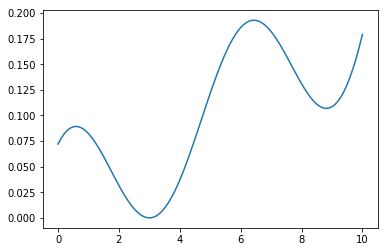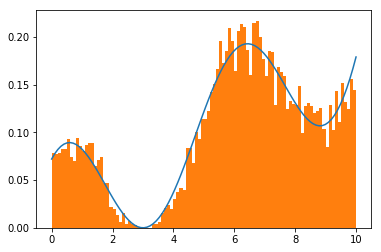Metropolis-Hastings算法（后面简称MH算法）是MCMC的代表算法，下面先对MH做一个简单的推导，然后写出MH算法流程，最后再对细节做讨论分析，本部分内容主要参考了刘建平：《MCMC(三)MCMC采样和M-H采样》

### 一.算法推导¶

$$p(x)p(x\rightarrow x')=p(x')p(x'\rightarrow x)$$

$$p(x)q(x\rightarrow x')\neq p(x')q(x'\rightarrow x)$$

$$p(x)q(x\rightarrow x')\alpha(x\rightarrow x')= p(x')q(x'\rightarrow x)\alpha(x'\rightarrow x)$$

$$\alpha(x\rightarrow x')=p(x')q(x'\rightarrow x)\\ \alpha(x'\rightarrow x)=p(x)q(x\rightarrow x')$$

#### 采样流程¶

（1）任意选择一个初始值$x_0$；

（2）对$i=1,2,...,n$循环执行：

（a）设状态$x_{i-1}=x$，按照建议分布$q(x\rightarrow x')$随机抽取一个候选状态$x'$；

（b）计算接收概率：

$$\alpha(x\rightarrow x')=p(x')q(x'\rightarrow x)$$

（c）从区间$(0,1)$中按均匀分布随机抽取一个数$u$，如果$u\leq \alpha(x\rightarrow x')$，则取状态$x_i=x'$，否则取$x_i=x$

（3）得到样本集合$\{x_{m+1},x_{m+2},...,x_n\}$

### 二.MH算法¶

MH算法的思想很朴实，那就是等比例的提升$\alpha(x\rightarrow x')$与$\alpha(x'\rightarrow x)$，但又不能超过1，这样既能保证细致平衡方程成立，又能提高接受率，举一例子来直观理解一下，比如我们在$(0,1)$上做均匀采样，接受率为0.1和接受率为1其实效果是一样的，采样结果最终都符合$(0,1)$上的均匀分布，但前者会拒绝掉90%的样本，那MH是怎么调整的勒，很简单，它分两种情况：

（1）若$p(x)q(x\rightarrow x')>p(x')q(x'\rightarrow x)$，那么，对等式两边同除以$p(x)q(x\rightarrow x')$：

$$1\cdot\alpha(x\rightarrow x')=\frac{p(x')q(x'\rightarrow x)}{p(x)q(x\rightarrow x')}\alpha(x'\rightarrow x)$$

$$\alpha(x\rightarrow x')=\frac{p(x')q(x'\rightarrow x)}{p(x)q(x\rightarrow x')}\\ \alpha(x'\rightarrow x)=1$$

（2）反过来，若$p(x')q(x'\rightarrow x)>p(x)q(x\rightarrow x')$，那么，对等式两边同除以$p(x')q(x'\rightarrow x)$：

$$\frac{p(x)q(x\rightarrow x')}{p(x')q(x'\rightarrow x)}\cdot\alpha(x\rightarrow x')=1\cdot\alpha(x'\rightarrow x)$$

$$\alpha(x\rightarrow x')=1\\ \alpha(x'\rightarrow x)=\frac{p(x)q(x\rightarrow x')}{p(x')q(x'\rightarrow x)}$$

$$\alpha(x\rightarrow x')=min\{1,\frac{p(x')q(x'\rightarrow x)}{p(x)q(x\rightarrow x')}\}\\ \alpha(x'\rightarrow x)=min\{1,\frac{p(x)q(x\rightarrow x')}{p(x')q(x'\rightarrow x)}\}$$

$$min\{1,\frac{p(x')q(x'\rightarrow x)}{p(x)q(x\rightarrow x')}\}>p(x')q(x'\rightarrow x)$$

#### MH采样流程¶

（1）任意选择一个初始值$x_0$；

（2）对$i=1,2,...,n$循环执行：

（a）设状态$x_{i-1}=x$，按照建议分布$q(x\rightarrow x')$随机抽取一个候选状态$x'$；

（b）计算接收概率：

$$\alpha(x\rightarrow x')=min\{1,\frac{p(x')q(x'\rightarrow x)}{p(x)q(x\rightarrow x')}\}$$

（c）从区间$(0,1)$中按均匀分布随机抽取一个数$u$，如果$u\leq \alpha(x\rightarrow x')$，则取状态$x_i=x'$，否则取$x_i=x$

（3）得到样本集合$\{x_{m+1},x_{m+2},...,x_n\}$

### 三.细致平衡方程校验¶

$$p(x\rightarrow x')=q(x\rightarrow x')\alpha(x \rightarrow x')$$

$$p(x)p(x\rightarrow x')=p(x')p(x'\rightarrow x)$$

$$p(x)p(x\rightarrow x')=p(x)q(x\rightarrow x')min\{1,\frac{p(x')q(x'\rightarrow x)}{p(x)q(x\rightarrow x')}\}\\ =min\{p(x)q(x\rightarrow x'),p(x')p(x'\rightarrow x)\}\\ =p(x')q(x'\rightarrow x)min\{1,\frac{p(x)q(x\rightarrow x')}{p(x')q(x'\rightarrow x)}\}\\ =p(x')q(x'\rightarrow x)$$

### 四.$q(x\rightarrow x')如何选择$¶

$q(x\rightarrow x')$比较省事儿的一种方法就是选择对称的建议分布，即：

$$q(x\rightarrow x')=q(x'\rightarrow x)$$

$$\alpha(x\rightarrow x')=min\{1,\frac{p(x')}{p(x)}\}$$

$q(x\rightarrow x')$通常可以选择：

（1）以$x$为均值，其协方差为常数矩阵的高斯分布；

（2）或者选择：

$$q(x\rightarrow x')\propto exp(-\frac{(x-x')^2}{2})$$

### 五.案例¶

$$p(x)=\frac{1}{1179}[(x-2)^2+(x-5)^3+100cos(x)+106],0<x<10$$

In :
import numpy as np
import matplotlib.pyplot as plt
%matplotlib inline

In :
def func(x):
#注意，超出范围的概率要设置为0
w=np.ones_like(x)
w=np.where(x<0,0,w)
w=np.where(x>10,0,w)
return w*((x-2)*(x-2)+(x-5)*(x-5)*(x-5)+100*np.cos(x)+106)/1179
x=np.linspace(0,10,100)
plt.plot(x,func(x))

Out:
[<matplotlib.lines.Line2D at 0x1be1ef9c320>]$$q(x,x')=\frac{1}{\sqrt{2\pi}}e^{-\frac{(x'-x)^2}{2}}$$

In :
#采样的样本量
nums=10000
count=0
points=[]
#采样x0
point=np.random.randn()
points.append(point)
while count<nums:
#按照q(x,x')，采下一个点：均值为0，方差为1的标准正态分布采样+point偏置
new_point=np.random.randn()+point
#alpha(x,x')
alpha=min(1.,func(new_point)/(1e-12+func(point)))
#从(0,1)均匀采样一个u
u=np.random.random()
#判断是否接收新点还是旧点
if u<alpha:
points.append(new_point)
point=new_point
else:
points.append(point)
count+=1

In :
#看看效果
plt.plot(x,func(x))
plt.hist(points,normed=True,bins=100)
plt.show()### 六.小结¶

In [ ]: